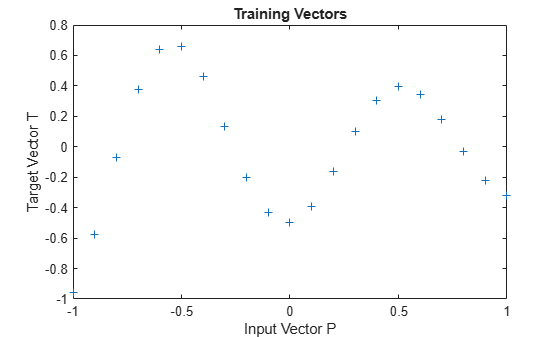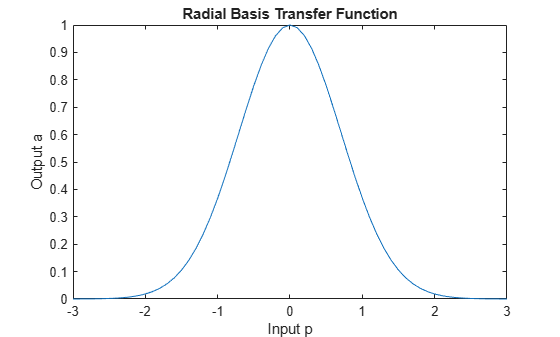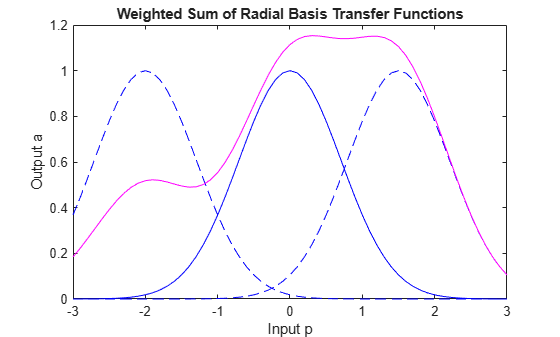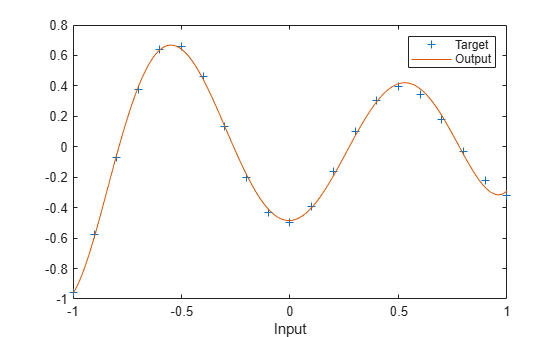Documentation

This example uses the NEWRB function to create a radial basis network that approximates a function defined by a set of data points.

Define 21 inputs P and associated targets T.

```X = -1:.1:1; T = [-.9602 -.5770 -.0729 .3771 .6405 .6600 .4609 ... .1336 -.2013 -.4344 -.5000 -.3930 -.1647 .0988 ... .3072 .3960 .3449 .1816 -.0312 -.2189 -.3201]; plot(X,T,'+'); title('Training Vectors'); xlabel('Input Vector P'); ylabel('Target Vector T');```We would like to find a function which fits the 21 data points. One way to do this is with a radial basis network. A radial basis network is a network with two layers. A hidden layer of radial basis neurons and an output layer of linear neurons. Here is the radial basis transfer function used by the hidden layer.

```x = -3:.1:3; a = radbas(x); plot(x,a) title('Radial Basis Transfer Function'); xlabel('Input p'); ylabel('Output a');```The weights and biases of each neuron in the hidden layer define the position and width of a radial basis function. Each linear output neuron forms a weighted sum of these radial basis functions. With the correct weight and bias values for each layer, and enough hidden neurons, a radial basis network can fit any function with any desired accuracy. This is an example of three radial basis functions (in blue) are scaled and summed to produce a function (in magenta).

```a2 = radbas(x-1.5); a3 = radbas(x+2); a4 = a + a2*1 + a3*0.5; plot(x,a,'b-',x,a2,'b--',x,a3,'b--',x,a4,'m-') title('Weighted Sum of Radial Basis Transfer Functions'); xlabel('Input p'); ylabel('Output a');```The function NEWRB quickly creates a radial basis network which approximates the function defined by P and T. In addition to the training set and targets, NEWRB takes two arguments, the sum-squared error goal and the spread constant.

```eg = 0.02; % sum-squared error goal sc = 1; % spread constant net = newrb(X,T,eg,sc);```
```NEWRB, neurons = 0, MSE = 0.176192 ```

To see how the network performs, replot the training set. Then simulate the network response for inputs over the same range. Finally, plot the results on the same graph.

```plot(X,T,'+'); xlabel('Input'); X = -1:.01:1; Y = net(X); hold on; plot(X,Y); hold off; legend({'Target','Output'})```